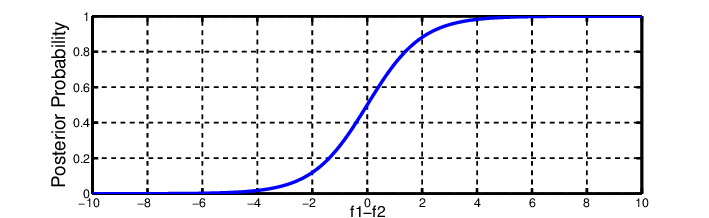/  Deep Learning Interview questions and answers   /  Explain Softmax Activation Function and difference between Sigmoid and Softmax Function## Explain Softmax Activation Function and difference between Sigmoid and Softmax Function

Ans: Softmax Function is mostly used in a final layer of Neural Network. Softmax Function not only maps our output function but also maps each output in such a way that the summation is equals to 1. In simple this function calculates the probability distribution of the events where these calculated probabilities determine the target class for the given inputs. It is implemented in final layer of Neural Network in order to classify the inputs into multiple categories.### Difference between Sigmoid and Softmax Function:

Sigmoid Function is used for Two class Logistic Regression. Sum of Probabilities need not to be 1. It is used as Activation Function while building Neural Networks. The high value will have the high probability but it need not to be the highest probability.Softmax Function is used for Multi class Logistic Regression. Sum of the Probabilities equals to 1. It is used in different layers of Neural Network. The high value will have the higher probability than others.